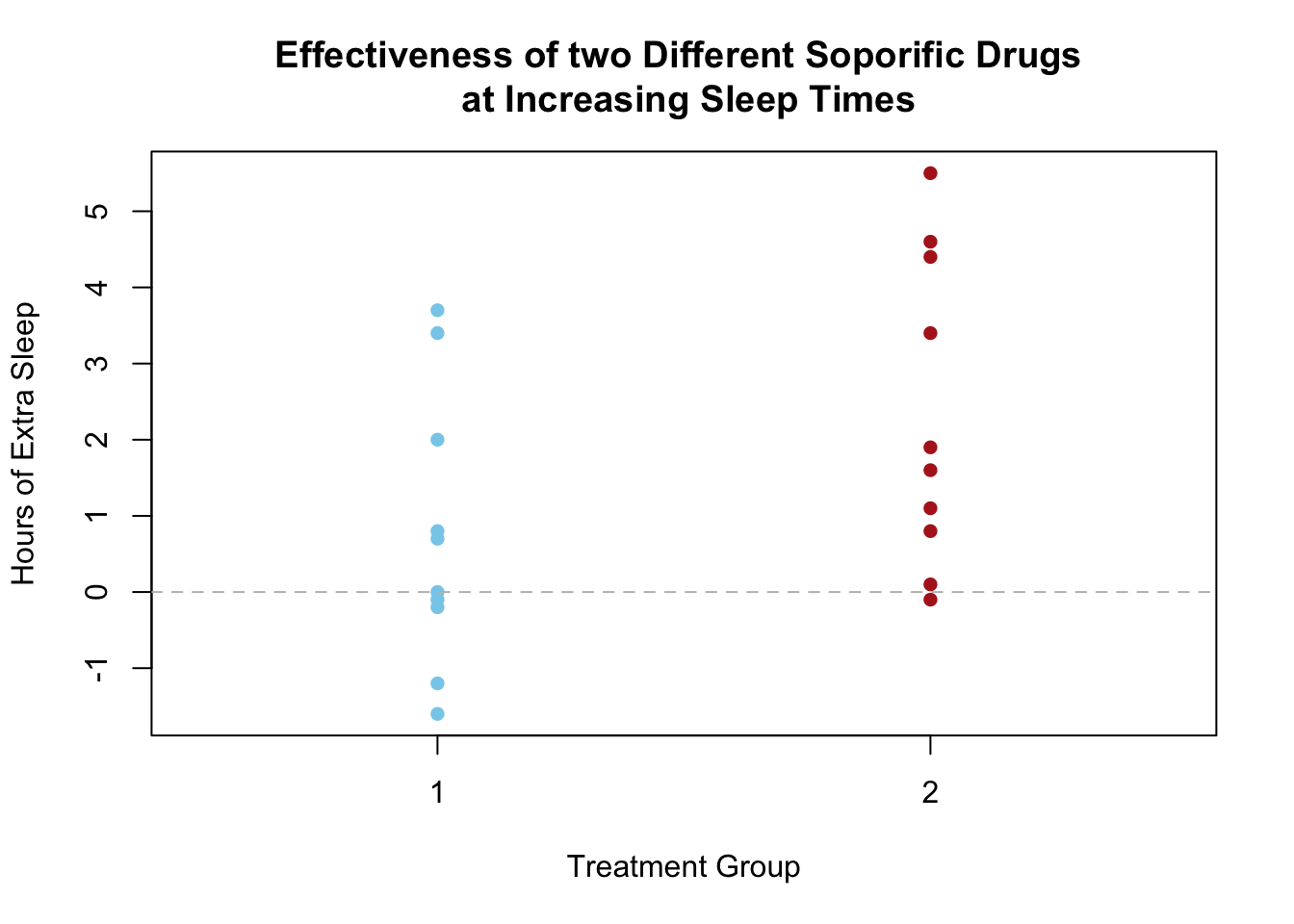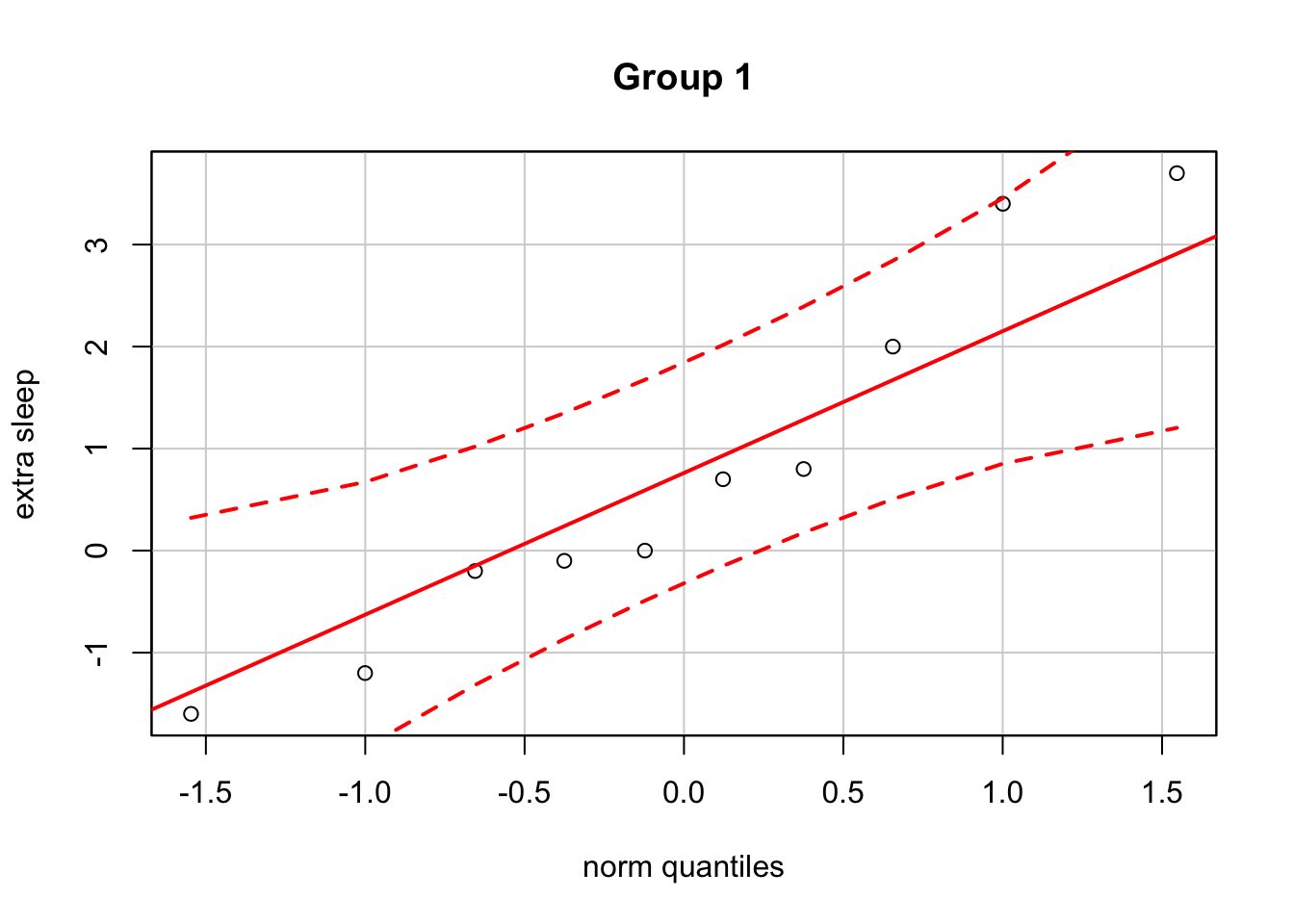#### Background

An experiment was conducted to determine which of two soporific drugs was better at increasing the hours of sleep individuals received, on average. There were two groups of 10 patients each. One group received the first drug, and the other group received the second drug. The amount of extra sleep that individuals received when drugged was measured. The data is contained in the sleep data set.

extra group ID
0.7 1 1
-1.6 1 2
-0.2 1 3
-1.2 1 4
-0.1 1 5
3.4 1 6
3.7 1 7
0.8 1 8
0.0 1 9
2.0 1 10
1.9 2 1
0.8 2 2
1.1 2 3
0.1 2 4
-0.1 2 5
4.4 2 6
5.5 2 7
1.6 2 8
4.6 2 9
3.4 2 10

It is assumed that the amount of extra sleep individuals will receive when using each drug is normally distributed. Thus, the interest is in knowing if $$\mu_1- \mu_2$$, the difference in the average hours of extra sleep received by individuals for each drug, is different from zero. In other words, is one drug better than the other at increasing the average hours of extra sleep.

Formally, the null and alternative hypotheses are written as $H_0: \mu_1 - \mu_2 = 0$ $H_a: \mu_1 - \mu_2 \neq 0$

The significance level for this study will be set at $\alpha = 0.05$

Note that the $$\neq$$ alternative hypothesis allows for either possibility, $$\mu_1 > \mu_2$$ or $$\mu_1 < \mu_2$$. If we selected a one-sided hypothesis, then only the stated alternative is considered possible, or of interest.

#### Analysis

The side-by-side dotplots of extra sleep demonstrate that the individuals in the study who took the second drug received more extra sleep on average than those taking the first drug. Also, notice that 9 out of 10 individuals taking the second drug experienced an increase in extra sleep. Of those taking the first drug, 5 out of 10 individuals experienced an increase in extra sleep. While these results are true for the individuals in the study, it is of interest to know if these results can be considered to hold generally in the population.

stripchart(extra ~ group, data=sleep, pch=16, col=c("skyblue","firebrick"),
vertical = TRUE, xlim=c(0.5,2.5), xlab="Treatment Group",
ylab="Hours of Extra Sleep", main="Effectiveness of two Different Soporific Drugs \n at Increasing Sleep Times")
abline(h=0, lty=2, col="gray")An independent samples t test could be used to test the previously stated null hypothesis. This will allow us to decide if the pattern in the sample data can be assumed to hold for the full population.

Before we can use an independent samples t test, the assumptions of the test must be shown to be satisfied. It is difficult to verify if the sampling distribution of $$\bar{x}_1 - \bar{x}_2$$ is normal. However, it is true that if the separate sampling distributions of $$\bar{x}_1$$ and $$\bar{x}_2$$ are normally distributed, then it follows that the sampling distribution of $$\bar{x}_1 - \bar{x}_2$$ will be normally distributed. As long as the population data is normal, it follows that the sampling distribution of the sample mean is normal.

qqPlot(sleep$extra[sleep$group==1], ylab="extra sleep", main="Group 1")qqPlot(sleep$extra[sleep$group==2], ylab="extra sleep", main="Group 2")